# IV.   Aircraft Performance

In this lecture we will make the connections between aircraft performance and propulsion system performance.

For a vehicle in steady, level flight, the thrust force is equal to the drag force, and lift is equal to weight.  Any thrust available in excess of that required to overcome the drag can be applied to accelerate the vehicle (increasing kinetic energy) or to cause the vehicle to climb (increasing potential energy).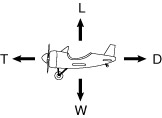Figure 4.1 Force balance for aircraft in steady level flight.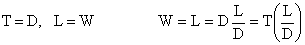## A.Vehicle Drag

Recall from fluids that drag takes the form shown below, being composed of a part termed parasitic drag that increases with the square of the flight velocity, and a part called induced drag, or drag due to lift, that decreases in proportion to the inverse of the flight velocity.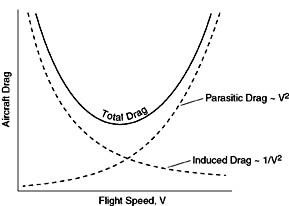Figure 4.2 Components of vehicle drag.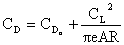where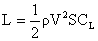and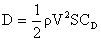Thus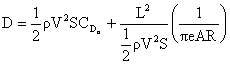orThe minimum drag is a condition of interest.  We can see that for a given weight, it occurs at the condition of maximum lift-to-drag ratio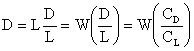We can find a relationship for the maximum lift-to-drag ratio by setting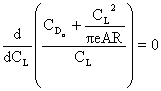from which we find that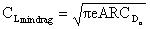and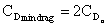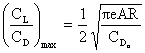and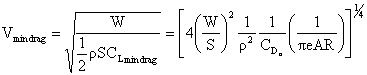## B.Power Required

Now we can look at the propulsion system requirements to maintain steady level flight since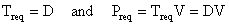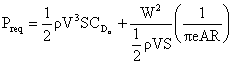Thus the power required (for steady level flight) takes the form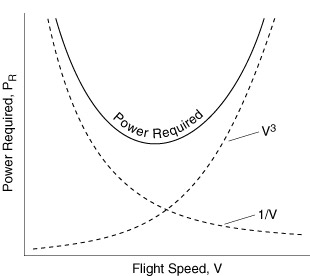Figure 4.3 Typical power required curve for an aircraft.

The velocity for minimum power is obtained by taking the derivative of the equation for Preq with respect to V and setting it equal to zero.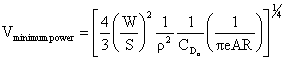As we will see shortly, maximum endurance (time aloft) occurs when the minimum power is used to maintain steady level flight.  Maximum range (distance traveled) is obtained when the aircraft is flown at the most aerodynamically efficient condition (maximum CL/CD).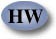## C. Aircraft Range, the Breguet Range EquationThe weight of the aircraft changes in response to the fuel burned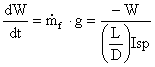or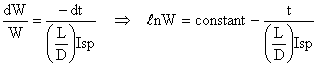applying the initial conditions,    at    t = 0         W = Winitial        \  const. = ln Winitial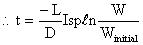the time the aircraft has flown corresponds to the amount of fuel burned, therefore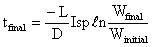then multiplying by the flight velocity we arrive at the Breguet Range Equation which applies for situations where Isp and flight velocity are constant over the flight.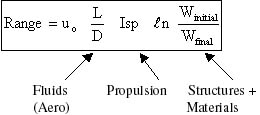This can be re-written in other forms: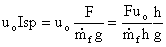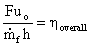where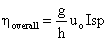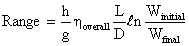or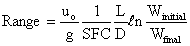## D.Aircraft Endurance

For a given amount of available fuel energy (Joules), the maximum endurance (time aloft) is obtained at a flight condition corresponding to the minimum rate of energy expenditure (Joules/second), or Preqmin, as shown in Figure 4.3.

We can determine the aerodynamic configuration which provides the minimum energy expenditure: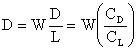so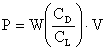where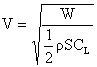Then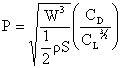So the minimum power required (maximum endurance) occurs when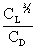is a maximum.

With a little algebra we can arrive at an expression for the maximum endurance.  Setting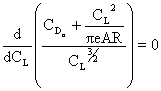we find that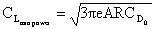and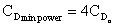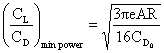and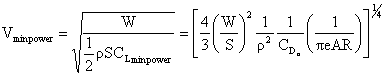Thus the minimum power (maximum endurance) condition occurs at a speed which is 3-1/4 = 76% of the minimum drag (maximum range) condition.  The corresponding lift-to-drag ratio is 86.6% of the maximum lift-to-drag ratio.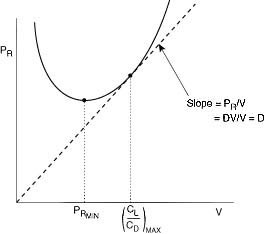Figure 4.4 Relationship between condition for maximum endurance and maximum range.

Continuing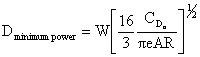which can be substituted into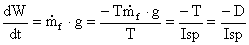Such that, for maximum endurance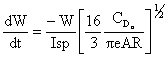which can be integrated (assuming constant Isp) to yield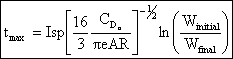## E.Climbing Flight

Any excess in power beyond that required to overcome drag will cause the vehicle increase kinetic or potential energy.  We consider this case by resolving forces about the direction of flight and equating these with accelerations.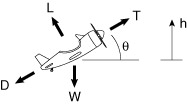Figure 4.5 Force balance for an aircraft in climbing flight.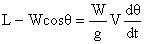where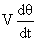is the accel. normal to the flight path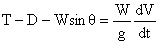where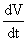is the accel. tangent to the flight path

So the change in height of the vehicle (the rate of climb, R/C) is: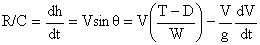which is instructive to rewrite in the form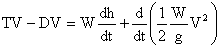or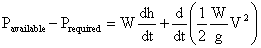in words:

excess power = change in potential energy + change in kinetic energy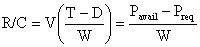and the time-to-climb is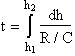wherefor example, and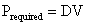The power available is a function of the propulsion system, the flight velocity, altitude, etc.  Typically it takes a form such as that shown in Figure 4.6.  The shortest time-to-climb occurs at the flight velocity where Pavail ­ Preq is a maximum.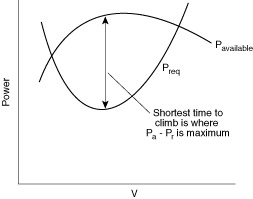Figure 4.6 Typical behavior of power available as a function of flight velocity.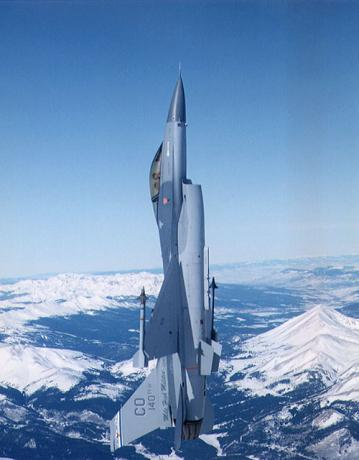Figure 4.7 Lockheed Martin F-16 performing a vertical accelerated climb.### To see more on climbing flight, visit NASA Glenn - GO!

 << Previous Unified Propulsion Next >>